Courses

# HC Verma Test: Work, Energy & Power

## 30 Questions MCQ Test Physics Class 11 | HC Verma Test: Work, Energy & Power

Description
This mock test of HC Verma Test: Work, Energy & Power for JEE helps you for every JEE entrance exam. This contains 30 Multiple Choice Questions for JEE HC Verma Test: Work, Energy & Power (mcq) to study with solutions a complete question bank. The solved questions answers in this HC Verma Test: Work, Energy & Power quiz give you a good mix of easy questions and tough questions. JEE students definitely take this HC Verma Test: Work, Energy & Power exercise for a better result in the exam. You can find other HC Verma Test: Work, Energy & Power extra questions, long questions & short questions for JEE on EduRev as well by searching above.
QUESTION: 1

### A ring of mass 200 gram is attached to one end of a light spring of force constant 100 N/m and natural length 10 cm. The ring is constrained to move on a rough wire in the shape of the quarter ellipse of the major axis 24 cm and the minor axis 16 cm with its centre at the origin. The plane of the ellipse is vertical and wire is fixed at points A and B as shown in the figure. Initially, ring is at A with other end of the spring fixed at the origin. If normal reaction of wire on ring at A is zero and ring is given a horizontal velocity of 10 m/s towards right so that it just reaches point B, then select the correct alternative (s) (g = 10 m/s2)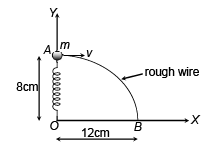Solution: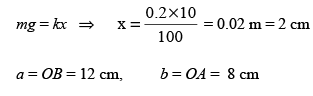∴ Spring is compressed by 2 cm at A and will be stretched by 2 cm at B.
By work-energy theorem,
Wmg + Ws + WN + Wf = Kf − Ki
0.16 + 0 + 0 + Wf = 0 - 10
Wf = -10.16

QUESTION: 2

### A block of mass m is stationary with respect to a wedge of mass M moving with uniform speed v on a horizontal surface. Find the work done by friction force on the block in t second.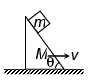Solution:

► Frictional force = mg sinθ
► Displacement = vt
► Work done = (mg sinθ) * (vt) * cos (π − θ) = - 1/2 mgvt sin2θ

QUESTION: 3

### A particle is kept at rest at the top of a sphere of diameter 42 m. When disturbed slightly, it slides down. At what height h from the bottom, the particle will leave the sphere?

Solution: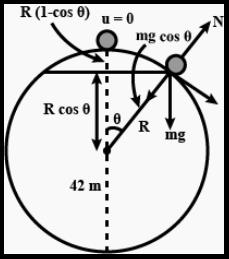Given:
D = 2R = 42m, R = 21m
h = R(1 − cosθ)
From the 3rd equation of motion,

v= u+ 2gh
v= 2gR(1−cosθ)  ...(1)

From the free body diagram: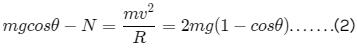When contact lost, N = 0, equation (2) becomes:

mgcosθ = 2mg(1−cosθ)
cosθ = 2−2cosθ
3cosθ = 2
cosθ = 2/3​

► 2R − h = 2R − R(1−cosθ)
► 2R − h = 2R − R(1−2/3​)
► 5R/3​ = 5/3 ​× 21 = 35m

QUESTION: 4

Inside a smooth hemispherical cavity, a particle P can slide freely. The block having this cavity is moving under constant acceleration A = g m/s2. A particle is released from the state of rest from the position shown. The angle θ with the vertical, when the particle will have a maximum velocity with respect to the block is: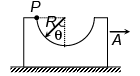Solution:

Velocity will be maximum w.r.t. block when net force w.r.t. block will become perpendicular to the relative velocity.
From FBD of the particle w.r.t. the cavity: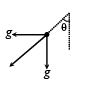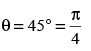QUESTION: 5

A uniform chain has a mass M and length L. It is placed on a frictionless table with length l0 hanging over the edge. The chain begins to slide down. The speed V with which the chain slides away from the edge is given by:

Solution:
• Since the given chain is uniform we can find the mass of the hanging part of the chain if we define some quantity lambda as mass per unit length and then multiplying it with the length of the hanging part of the chain.
• Also, while applying the energy conservation equation for extended bodies (like chain, etc) we need to consider the energies (both kinetic and potential) of the center of mass only. We will consider the dotted line as a reference line while applying energy equation.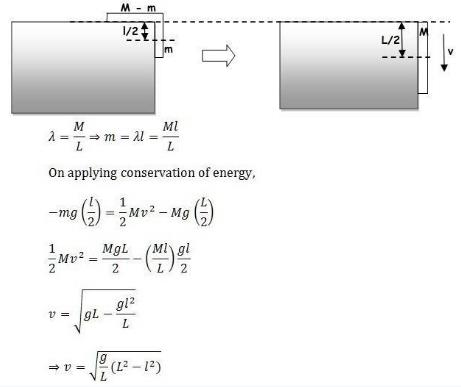QUESTION: 6

Discs, each having mass m, are attached rigidly to the ends of a vertical spring. One of the discs rests on a horizontal surface and the other produces a compression x0 on the spring when it is in equilibrium. How much further must the spring be compressed so that when the force causing compression is removed, the extension of the spring will be able to lift the lower disc off the table?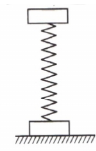Solution:

Now for lifting up of the lower disc: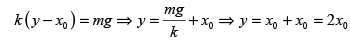QUESTION: 7

A particle is dropped from a height h. A constant horizontal velocity is given to the particle.
Taking g to be constant every where, kinetic energy E of the particle with respect to time t is correctly shown in

Solution:
• As the particle is projected with some velocity, therefore, its initial kinetic energy will not be zero.
• As it moves downward under gravity then its velocity increases with time K.E. ∝ v2 ∝ t2 (As υ ∝ t)
• So the graph between kinetic energy and time will be parabolic in nature.
QUESTION: 8

A pendulum of mass m and length l is suspended from the ceiling of a trolley which start with a constant acceleration a in the horizontal direction as shown in figure. The maximum angular displacement of pendulum is θ. Work done by the tension w.r.t. trolley in this time interval is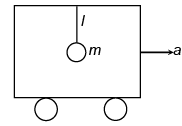Solution:
• Work done by tension is zero because T perpendicular to the displacement.

The free-body diagram of the pendulum with respect to the trolley is shown in figure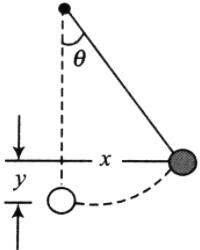Free-body diagram of the pendulum bob with respect to trolley.
The forces acting on the bob are:

• the gravity, mg
• the pseudo force, ma
• the tension, T

The work done by gravity, Wg = -mgl (1 − cosθ) = −mgl [2 sin2 (θ/2)]

The work done by pseudo force, Wps = ma.l sinθ

The work done by tension, Wt = 0

At the position of maximum deflection, the velocity of the bob is zero, ΔK=0ΔK=0.

Applying work-energy theorem, we get
Wg+Wps+WT=ΔK
−mg[2 sin2 (θ/2)]+ma[2sin (θ/2) cos (θ/2)] = 0
tan (θ/2) = ag or θ=2 tan−1(ag)

QUESTION: 9

A block of mass m is attached to two unstretched springs of spring constants k 1 and k 2 as shown in figure. The block is displaced towards right through a distance x and is released. Find the speed of the block as it passes through the mean position shown.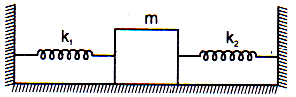Solution: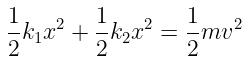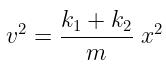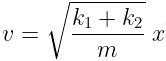QUESTION: 10

A particle moves on a rough horizontal ground with some initial velocity say v0. If (3/4)th of its kinetic energy is lost due to friction in time t0 then coefficient of friction between the particle and the ground is

Solution:

3/4 th energy is lost i.e. 1/4 th kinetic energy is left.

Hence its velocity  becomes v0/2 under a retardation of μg in time t0.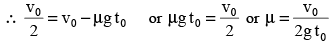QUESTION: 11

A block weighing 10 N travels down a smooth curved track AB joined to a rough horizontal surface (figure). The rough surface has a friction coefficient of 0.20 with the block. If the block starts slipping on the track from a point 1.0 m above the horizontal surface, the distance it will moves on the rough surface is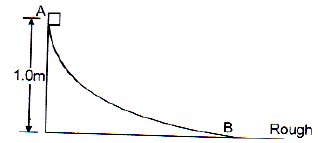Solution:

Let the velocity at point B be v.

By conservation of energy, mgh = (1/2 ) mv⇒ v = √(2gh​)
Now deceleration due to friction is a = μg = 0.2 g
So stopping distance, s = v2/(2a) ​= (2gh) / (0.4g) = 5 h = 5 × 1 = 5m

QUESTION: 12

A small block of mass m is lying at rest at point P of a wedge having a smooth semi circular track of radius R. The minimum value of horizontal acceleration a0 of wedge so that mass can just reach the point Q ?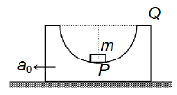Solution:

On applying work energy theorem in the frame of wedge.

ma0 R − mgR = 0 ⇒ a0 = g

QUESTION: 13

A wedge of mass M is fitted with a spring of stiffness ‘k’ is kept on a smooth horizontal surface. A rod of mass m is kept on the wedge as shown in the figure. System is in equilibrium.
Assuming that all surfaces are smooth, the potential energy stored in the spring is: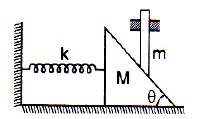Solution:

N sinθ = kx
N cosθ = mg
We get the value of x,
x = (mg tanθ) / k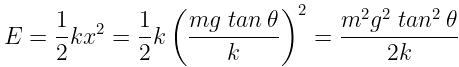QUESTION: 14

A body of mass m tied at the end of a massless string of length l (fixed at other end) is projected with horizontal velocity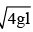. At what height from initial level will it leave
the circular path?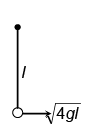Solution:

The height at which body leave the circle.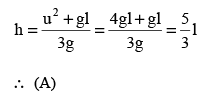QUESTION: 15

The potential energy of a particle varies with a distance x as shown in the graph.The force acting on the particle is zero at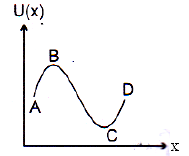Solution:

The slope of the energy distance graph represents force.

∵ At points B, C slope is zero,
Therefore, force acting is also zero

QUESTION: 16

The ends of a spring are attached to blocks of masses 3 kg and 2 kg. The 3 kg block rests on a horizontal surface and the 2 kg block which is vertically above it is in equilibrium producing a compression of 1 cm of the spring. The value of the length to which the 2 kg mass must be compressed, so that when it is released, the 3 kg block may be lifted off the ground is: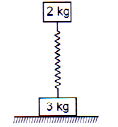Solution:

We have two blocks with upper block in equilibrium. The upper block compresses the spring by 1 cm.

Therefore force by spring is equal to the weight of 2 kg block.
kx = 2g ⇒ k * 1 = 2g ⇒ k = (2g)/1 = 20 N/cm = 2000 N/m

Now let the total compression after exerting force be y. When the force is removed the upper block goes up lifting the lower block upward.
At this time the normal reaction force acting on the body becomes zero.
This means spring force will be equal to the sum of the weights of upper block and lower block.

Ky = 2g + 3g
Replacing k by 2000N/m and g by 10m/s2
2000 y = 2 * 10 + 3 * 10 = 50
⇒ y = 50/2000
⇒ y = 0.025 m = 0.025 x 100
⇒ y = 2.5 cm

QUESTION: 17

A block of mass m is placed on an another rough block of mass M and both are moving horizontally with same acceleration a due to a force which is applied on the lower block, then work done by lower block on the upper block in moving a distance s will be

Solution:

Force on the upper block = ma Work done = F.S = mas

QUESTION: 18

A body of mass m accelerates uniformly from rest to a speed v0 in time t0 . The work done on the body till any time t is

Solution: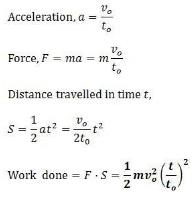QUESTION: 19

An inclined plane ends into a vertical loop of radius r as shown in figure. If a particle of mass m is released from topmost point. The point C on the track as shown in the figure and centre of circular track are at same horizontal level. The normal force at point C is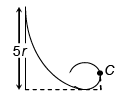Solution:

velocity at point C is v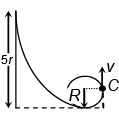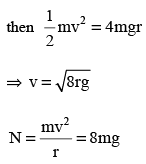QUESTION: 20

In the system shown in the figure there is no friction anywhere. The block C goes down by a distance x0 = 10 cm with respect to wedge D when system is released from rest. The velocity of A with respect to B will be ( g = 10 m/s2 )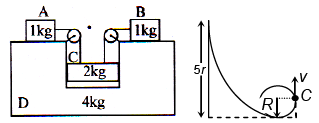Solution:
QUESTION: 21

A uniform rod of length L and mass M is placed on a rough horizontal surface. The force F applied on the rod is such that the rod is just in the state of rest. If the coefficient of friction varies according to the relation μ = KX, where K is a positive constant. Suppose friction is present only from X = 0 to X = L. If the rod moves in the direction of application of force then the total heat generated in the time in which the rod crosses this region, is equal to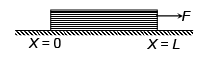Solution: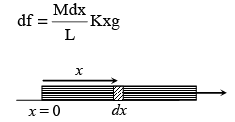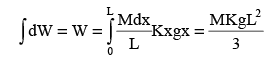QUESTION: 22

A block of mass 1 kg is kept over another block of mass 2 kg and whole system is kept on a smooth X- Z plane. Block of mass 1 kg is being moved continuously with velocity 1 m/s towards positive X- axis and lower block is moved continuously with velocity 1 m/s towards positive Z- axis as shown in figure. If coefficient of friction between two blocks is 0.1, the rate at which heat is generated will be (g = 10 m/s2)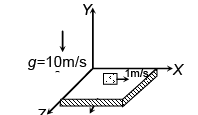Solution: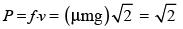QUESTION: 23

In the figure shown all the surfaces are frictionless, and mass of the block, m = 1 kg. The block and wedge are held initially at rest. Now wedge is given a horizontal acceleration  of 10 m/s2 by applying a force on the wedge, so that the block does not slip on the wedge. Then work done by the normal force in ground frame on the block in √3 seconds is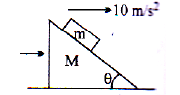Solution:

Let the Normal Reaction of Wedge on the Block be 'N'.

We know,
Acceleration of Block , a = 10 m/s2
Initial velocity of block , u = 0 m/s

Using Newton's Equation of motion,
T=√3s
S = ut + (1/2) at2 = 0 + (1/2) x 10 x (√3)= 15

Work done by the Normal Force,W= N x S x cos(π/2- θ)
= N x 15 x sin(θ) = 10 x 15 = 150N

Hence, Work Done by the Normal Force in the ground Frame on the block is 150 N.

QUESTION: 24

A block of mass 1 kg starts moving at t = 0 with velocity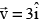on a rough horizontal platform (with coefficient of friction μ = 0.3) moving with velocity 4ˆj . At t = t0 block comes to rest with respect to platform. Then displacement of block with respect to ground in this time interval. (x- y plane is a horizontal plane, g = 10 m/s2)

Solution:

FBD wrt Platform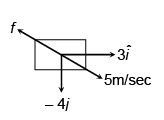Time after which block comes to rest relative to platform = 5/3 sec.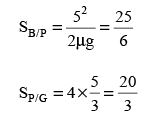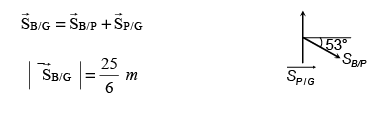QUESTION: 25

Suppose potential energy between particle A and B at separation r is given by U = k ln r, where k is constant. For such hypothetical system, the ratio of energy difference between energy levels (n = 1 and n = 2) and (n = 2 and n = 4) is (The energy of a particle in nth level is –13.6/n2)

Solution: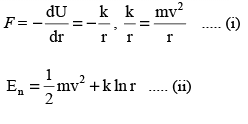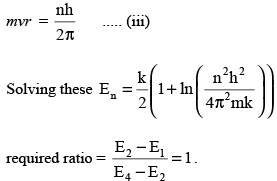QUESTION: 26

A 1.0 kg block collides with a horizontal weightless spring of force constant 2.75 Nm−1 as shown in figure. The block compresses the spring 4.0 m from the rest position. If the coefficient of kinetic friction between the block and horizontal surface is 0.25, the speed of the block at the instant of collision is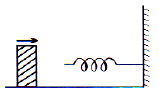Solution:

Work done on m=change in kinetic energy
⇒ work done by friction and PE energy in spring= Δk.E
⇒ μn(x)+1/2kx2=1/2mv2
⇒ 0.25 x 10 x 4 + 1/2 x 275/100 x 16 = 1/2 x (1) v2
⇒10+22=1/2v2
⇒ 32x2=v2
⇒ 64=v2
⇒ v=8m/s

QUESTION: 27

Three blocks A, B and C are kept as shown in figure. The coefficient of friction between A and B is 0.2, B and C is 0.1, C and ground is 0.0. The mass of A, B and C are 3 kg, 2 kg and 1 kg respectively. A is given a horizontal velocity 10 m/s. A, B and C always remain in contact i.e. lies as in figure. The total work done by friction will be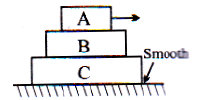Solution:

The block A is given 10m/s velocity
Initial momentum of the system = 3 x 10 = 30
As no external force is applied the linear momentum of the system: 30=6xv
v=5 m/s
The final mass will be taken 6 kg as it is given that finally, all the blocks are moving together as a system.

By the conversation of energy
(1/2) x 3 x 10+ frictional work done = (1/2) x 6 x 52
⇒ Frictional work done = -75J

QUESTION: 28

A block of mass m is placed inside a smooth hollow cylinder of radius R kept horizontally.Initially system was at rest. Now cylinder is given constant acceleration 2 g in the horizontal direction by external agent. The maximum angular displacement of the block with the vertical is: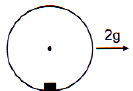Solution:

work done by acc of sphere = m*2gR sin∅
work done by gravity = - m*gR(1 - cos ∅)
∆KE = 0
using work-energy threorm
2 mgR sin ∅ - mgR(1 - cos ∅) = 0
2 mgR sin ∅ = mgR(1 - cos ∅)
2 sin ∅ = (1 - cos ∅)
∅ = 2 tan-12

QUESTION: 29

Two point masses of mass 4 m and m respectively separated by a distance d are revolving under mutual force of attraction. Ratio of their kinetic energy will be

Solution: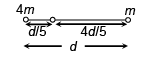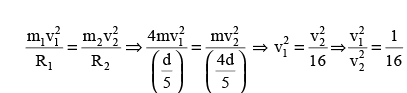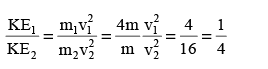QUESTION: 30

When an object is allowed to slide down a hill it stops at the point B because of friction. If friction force depends only on the normal component of the reaction force with coefficient of friction variable along the path, the work done in taking the object slowly from B to A along the hill will be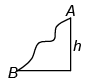Solution:

Work done by friction down the plane = – mgh

work done in taking the object from B to A = 2 mgh